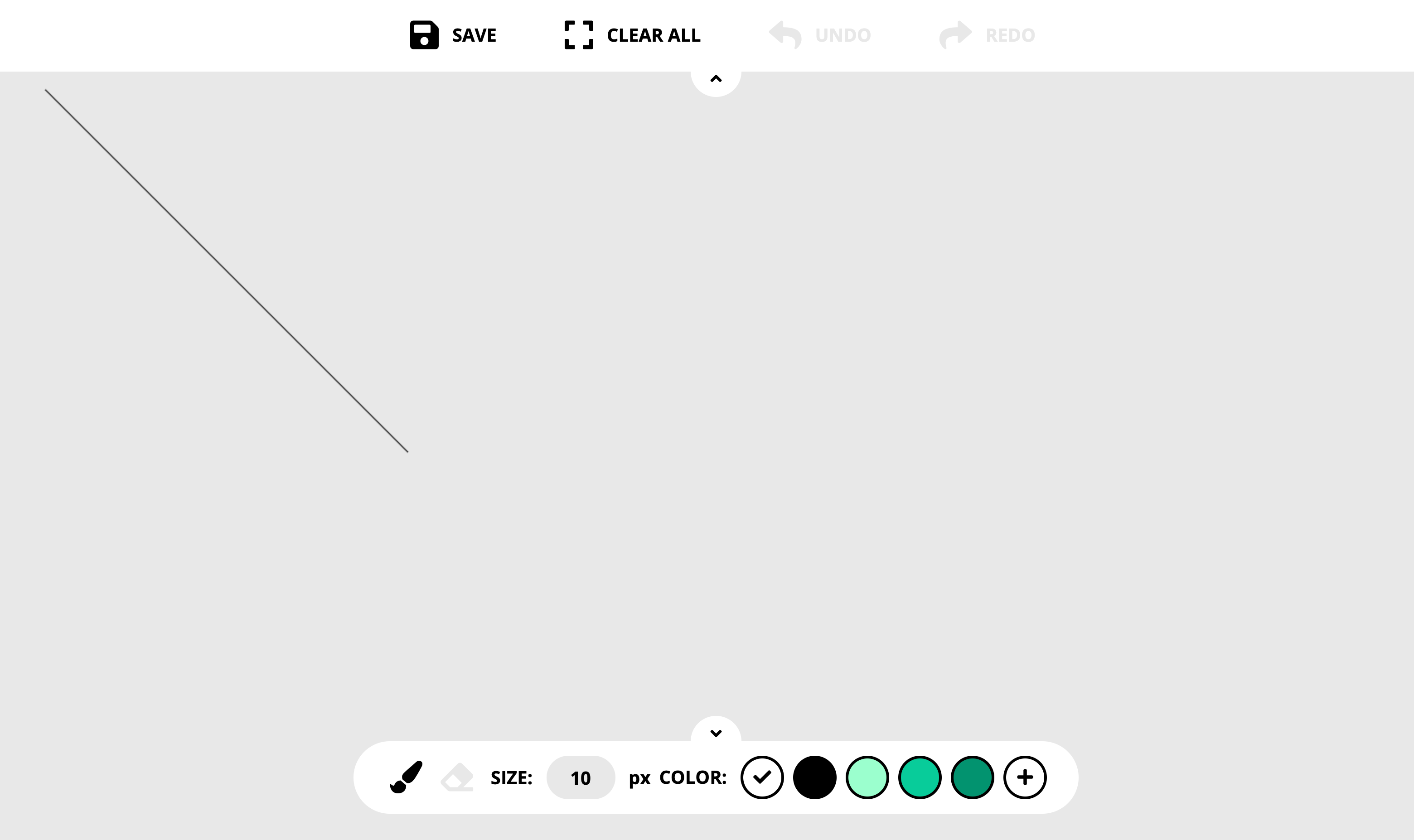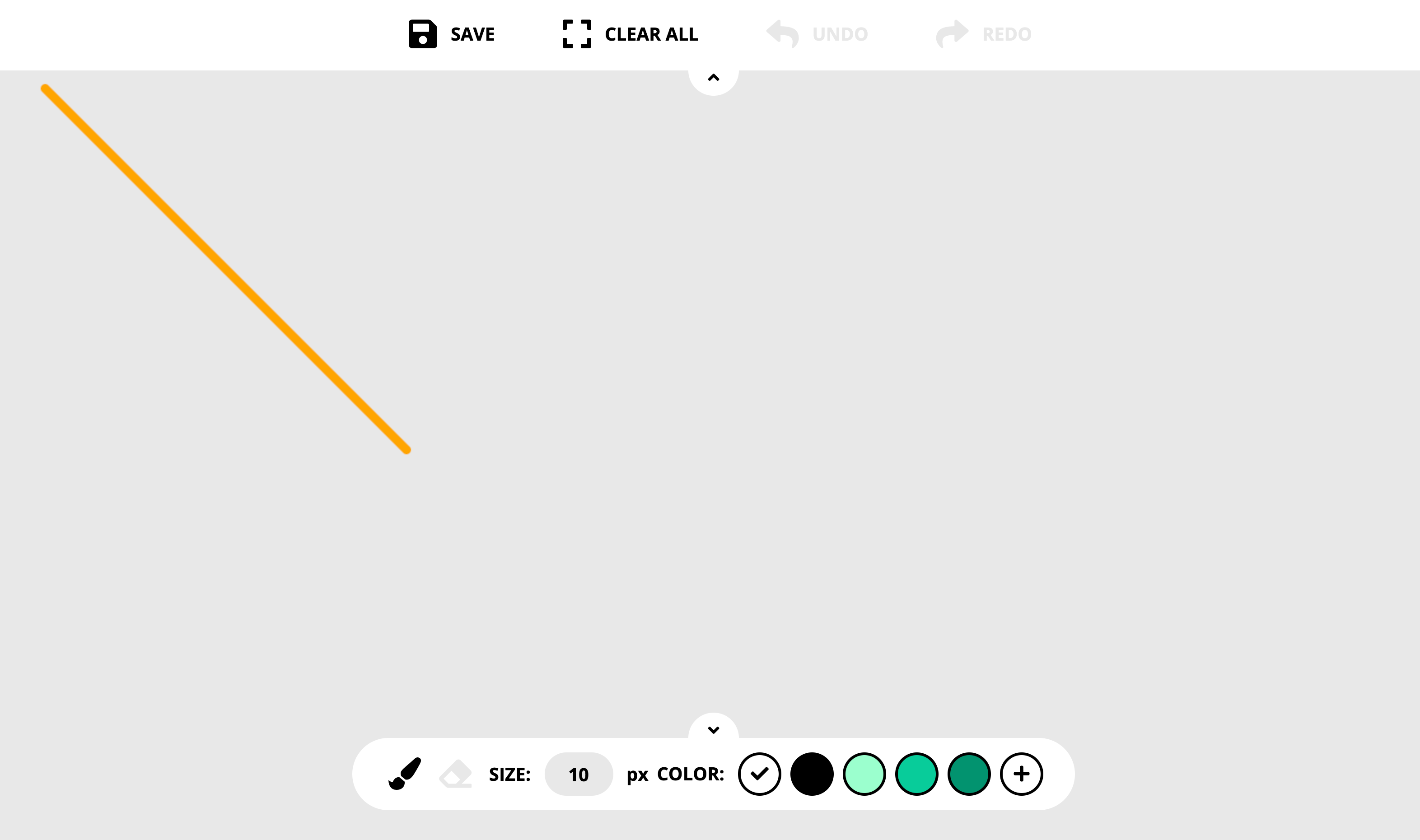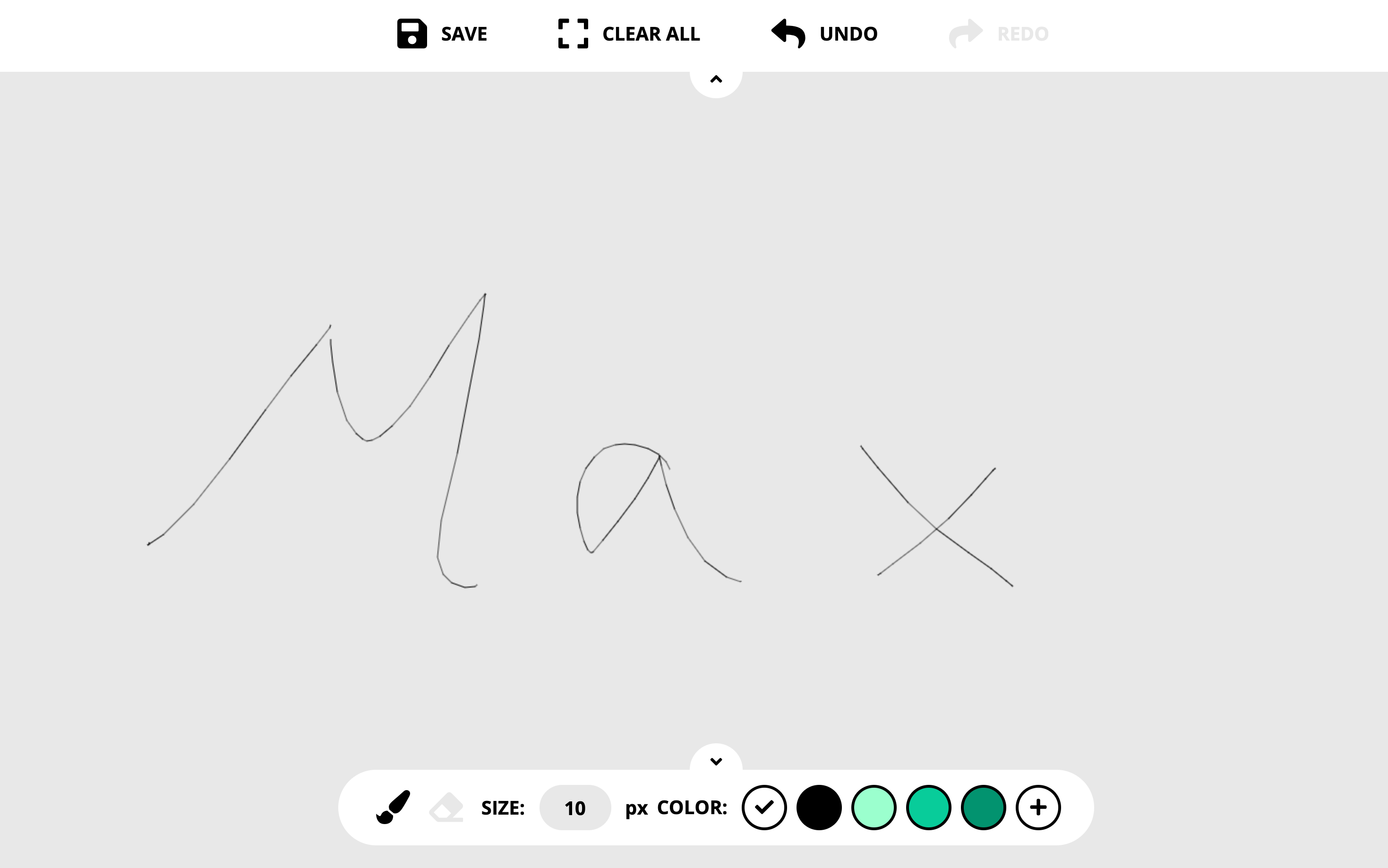# JS地下城 - Canvas

## 確認需求

• 讓使用者可以使用滑鼠在畫布上繪圖
• 畫筆要可以進行基本設定(粗細、顏色)
• 一些畫布的基本功能(清空、復原、重做)
• 能夠將畫作下載為圖檔

## 解題攻略

#### # 準備畫布

``<canvas id="draw"></canvas>``
``````let canvas = document.querySelector("#draw");
let ctx = canvas.getContext("2d");``````

``````function setSize() {
let canvasWidth = window.innerWidth();
let canvasHeight = window.innerHeight();
canvas.setAttribute("width", canvasWidth);
canvas.setAttribute("height", canvasHeight);
}``````

``````// 開始繪圖
ctx.beginPath();
// 設定起始座標
ctx.mobeTo(x, y);
// 設定終點座標
ctx.lineTo(x, y);
// 繪製
ctx.stroke();``````#### # 畫筆設定

``````ctx.strokeStyle = "#FFA500";
ctx.lineWidth = 10;
ctx.lineCap = "round";``````#### # 繪畫互動

``````let lastPointX, lastPointY;

let downHandler = function(e){
// 滑鼠按下去時得到座標存在變數中作為等等畫圖的起點
lastPointX = e.offsetX;
lastPointY = e.offsetY;
// 並且為Canvas綁定mousemove和mouseup的事件
};

let moveHandler = function(e) {
// 滑鼠在移動時我們把新的座標存下來作為終點
let newPointX = e.offsetX;
let newPointY = e.offsetY;

// 畫圖四步驟
ctx.beginPath();
ctx.moveTo(lastPointX, lastPointY);
ctx.lineTo(newPointX, newPointY);
ctx.stroke();

// 把終點改為新的起點
lastPointX = newPointX;
lastPointY = newPointY;
};

let upHandler = function(){
// 滑鼠釋放後把剛剛綁定的事件移除
draw.removeEventListener("mousemove", moveHandler);
draw.removeEventListener("mouseup", upHandler);
};#### # 進階功能

• Path2D Object 是利用 `MyPath = new Path2D()` 來建立一個路徑物件，可以事先存取路徑再利用 `ctx.stroke(MyPath)` 畫出來，但這個物件只能存取路徑卻無法存取畫筆顏色和樣式。
• `save()` `restore()` 可以存取畫布狀態並重新呼叫，但一次只能存取一個狀態到 stack 中，看來也不是我們需要的。

``````let step = -1;
let history = [];

let push = function(){
step++;
if (step <= history.length - 1) history.length = step
history.push(canvas.toDataURL())
}

// 記得將push()加入upHandler中``````

``````A -> B -> C -> D   step = 3
// 我們總共畫了四筆，分別都存進 history[A,B,C,D]

A -> B -> C   [D]  step = 2
// 我們發現Ｄ畫錯了，所以復原到Ｃ，但Ｄ仍然是 history 裡的第步

A -> B -> C -> E  [D̶] step = 3
// 這時候我們重畫了一個Ｅ，它是新的第步，而舊的Ｄ必須被我們覆蓋掉
// history[A,B,C,E]``````

``````let undo = function(){
// 創建一個新的圖像物件
let lastDraw = new Image;

// 確定有上一步我們才回到上一步
if(step > 0) step--;

// 把上一部的base64設定給圖像物件
lastDraw.src = history[step];

// 把圖片載入後用畫布渲染出來
ctx.clearRect(0, 0, canvasWidth, canvasHeight);
ctx.drawImage(lastDraw, 0, 0);
};
};``````

#### # 保存作品

``````let save = document.querySelector("#save");

})``````

## 加分功能

``let brushColor = ["#ffffff","#000000","#9BFFCD","#00CC99","#01936F"];````````let isDark = function(color) {
let rgbArray = [color.substr(1,2), color.substr(3,2), color.substr(5,2)];

let brightness =
parseInt(`0x\${rgbArray}`) * 0.213 +
parseInt(`0x\${rgbArray}`) * 0.715 +
parseInt(`0x\${rgbArray}`) * 0.072

return brightness < 255 / 2
}``````